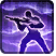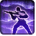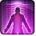Imperial PreparationConditions

Used by

Used by
• apc.agent.sniper.base

Related effects, buffs and debuffs

Please click on an effect below to view its details.

• [0s] [not displayed]Effect #1

 Slot: Buff Duration: 0s Tick rate: does not tick # occurrences: 0
• On Apply

Perform the following actions:

• Play appearance epp . agent . imperial_preparation . cast_instant
• Modify Cooldown
- Is Percentage = (bool) true
- Tag = (string)
- Tags = (string)
- Ability Spec = (int) 16141019349800161796
- Amount Min = (float) 0
- Amount = (float) -1
• Modify Cooldown
- Is Percentage = (bool) true
- Tag = (string)
- Tags = (string)
- Ability Spec = (int) 16140925134370843524
- Amount Min = (float) 0
- Amount = (float) -1
• Modify Cooldown
- Is Percentage = (bool) true
- Tag = (string)
- Tags = (string)
- Ability Spec = (int) 16140997487124955457
- Amount Min = (float) 0
- Amount = (float) -1
• Modify Cooldown
- Is Percentage = (bool) true
- Tag = (string)
- Tags = (string)
- Ability Spec = (int) 16140967652545713457
- Amount Min = (float) 0
- Amount = (float) -1
• Modify Cooldown
- Is Percentage = (bool) true
- Tag = (string)
- Tags = (string)
- Ability Spec = (int) 16141092238594645230
- Amount Min = (float) 0
- Amount = (float) -1
• Modify Cooldown
- Is Percentage = (bool) true
- Tag = (string)
- Tags = (string)
- Ability Spec = (int) 16141009928756419818
- Amount Min = (float) 0
- Amount = (float) -1
• [15s]Over-prepared
Damage reduction increased.

 Slot: Buff Duration: 15s Tick rate: does not tick # occurrences: 1 Conditions: If TARGET has the ability 16141137256899023541 – not found, with a rank >= 1
• On Apply

Perform the following actions: